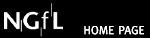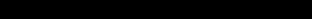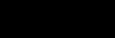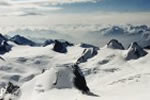## River Discharge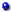IntroductionCalculation

The discharge of a river is the volume of water which flows through it in a given time. It is usually measured in cubic meters per second.
The volume of the discharge will be determined by factors such as climate, vegetation, soil type, drainage basin relief and the activities of man.

Discharge is very easy to calculate, being the cross-sectional area of the channel multiplied by the velocity of the water.

However, before you relax, finding the cross-sectional area and average velocity can take some time and not a little effort. Firstly, let's look at finding the easier of the two values, the average velocity.

Average velocity

The velocity of the water within a river channel varies according to depth and nearness to obstacles such as the bed, banks and boulders which generate friction and drag. If you have recorded the surface velocity, a good estimate of the average can be found by multiplying your surface value by 0.8. For example, a surface value of 3 meters per second would be converted thus...

```
Measured surface value x 0.8 = average velocity

3.0ms x 0.8 = 2.4 ms

```
If you have carried out a detailed survey of velocity throughout the cross-section, using a velocity meter ( flow vane ) you can plot your readings on graph paper and work out the average velocity from that.

Either way, don't forget to ensure that for the final discharge reading you use the correct units for area and time - meters and seconds.

```

```
Cross-sectional area

Now for the cross-sectional area. Having recorded all your depth data in the field you will probably be intending to plot it on graph paper to produce a cross-section diagram. Once this has been drawn it is possible to find the area simply by counting the number of squares in the 'wet' part of the diagram. This works quite well for small streams but the number of squares on the graph paper rapidly goes into the hundreds and even thousands. Keeping an accurate record of which ones you have counted is difficult without marking them on the actual diagram. This may not be desirable if you want to display the diagram afterwards.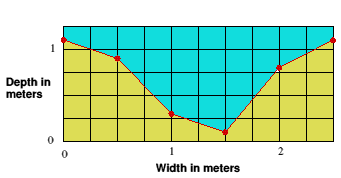Count the grid squares or divide the diagram into squares
and triangles to find the area

An alternative is to break up the cross-section in to triangles and rectangles, finding the area of each and then adding them together.